# NumPy Tensors, Slicing, and Images¶

Here's a more detailed example of how to interpret images as NumPy tensors.

# Tensors¶

(D = ndim)# 2D Color Image = 3D Tensor¶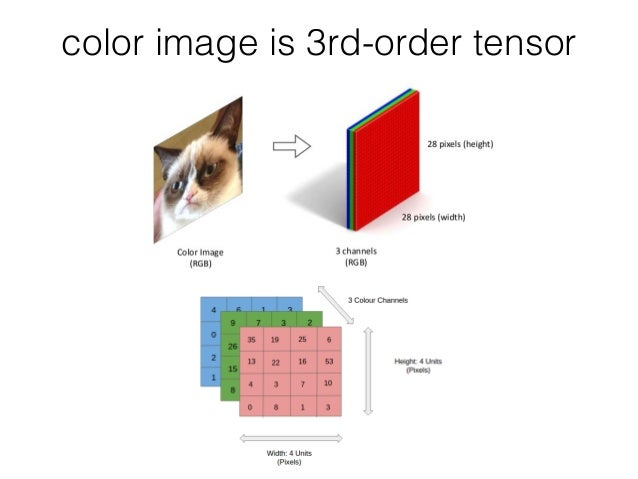# Images and Videos: 2D, 3D, 4D, 5D¶

Image type Coordinates
2D grayscale image (row, col)
2D color image (eg. RGB) (row, col, channel)
3D grayscale image (plane, row, col)
3D color image (plane, row, col, channel)
Video type Coordinates
2D color video (time, row, col, ch)
3D multichannel video (time, plane, row, col, ch)

# A simple 3D tensor¶

In [ ]:
import numpy as np

t = np.array([[
[1, -1, 11, -11],
[2, -2, 22, -22]
],

[
[3, -3, 33, -33],
[4, -4, 44, -44]
],

[
[5, -5, 55, -55],
[6, -6, 66, -66]
]])
t

In [ ]:
t.ndim # how many dimensions

In [ ]:
t.shape # what the dimensions are


### Tensor Lego¶

Here's a Lego representation of our tensor.

• Each 8-peg Lego brick represents one element.
• There are 3 major blocks
• Each major block contains 2 levels, each level has 4 elements.
• The flat gray piece will be our "slicer"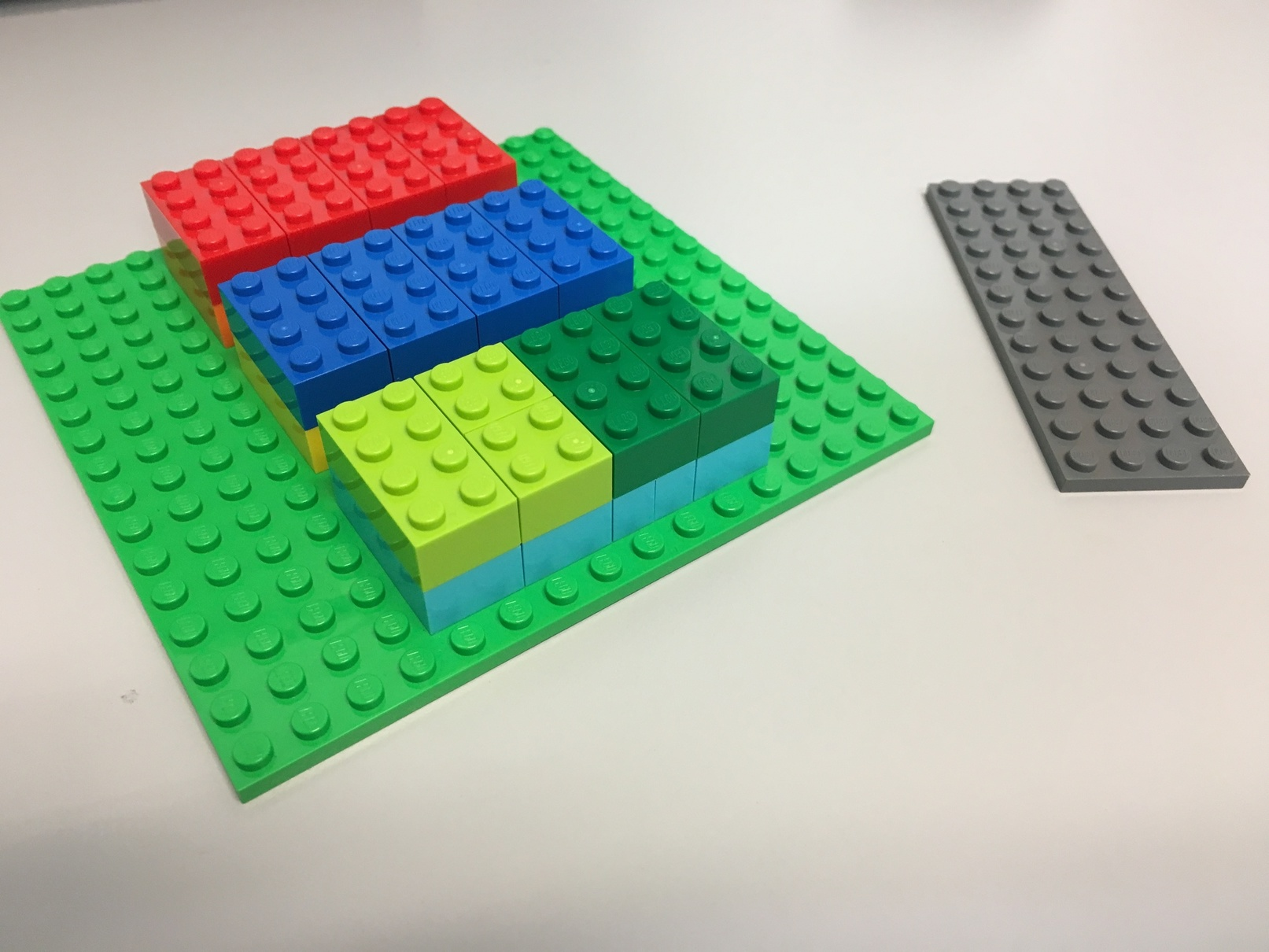### Terminology and Rules¶

• By array, we refer to NumPy arrays (np.array), which can be multi-dimensional.

• Given an array of N dimensions, a slice always returns an array of N-1 dimensions.

### Slicing along the first dimension¶

The first dimension views our tensor this way:


t = np.array([
first_level_array0,  # [[  1,  -1,  11, -11],
#  [  2,  -2,  22, -22]]  <-- note the double square brackets
surrounding each chunk [[ ]]

first_level_array1,  # [[  3,  -3,  33, -33],
#  [  4,  -4,  44, -44]]

first_level_array2   # [[  5,  -5,  55, -55],
#  [  6,  -6,  66, -66]]
])

Therefore, a slice along the first dimension will cut in between the chunks: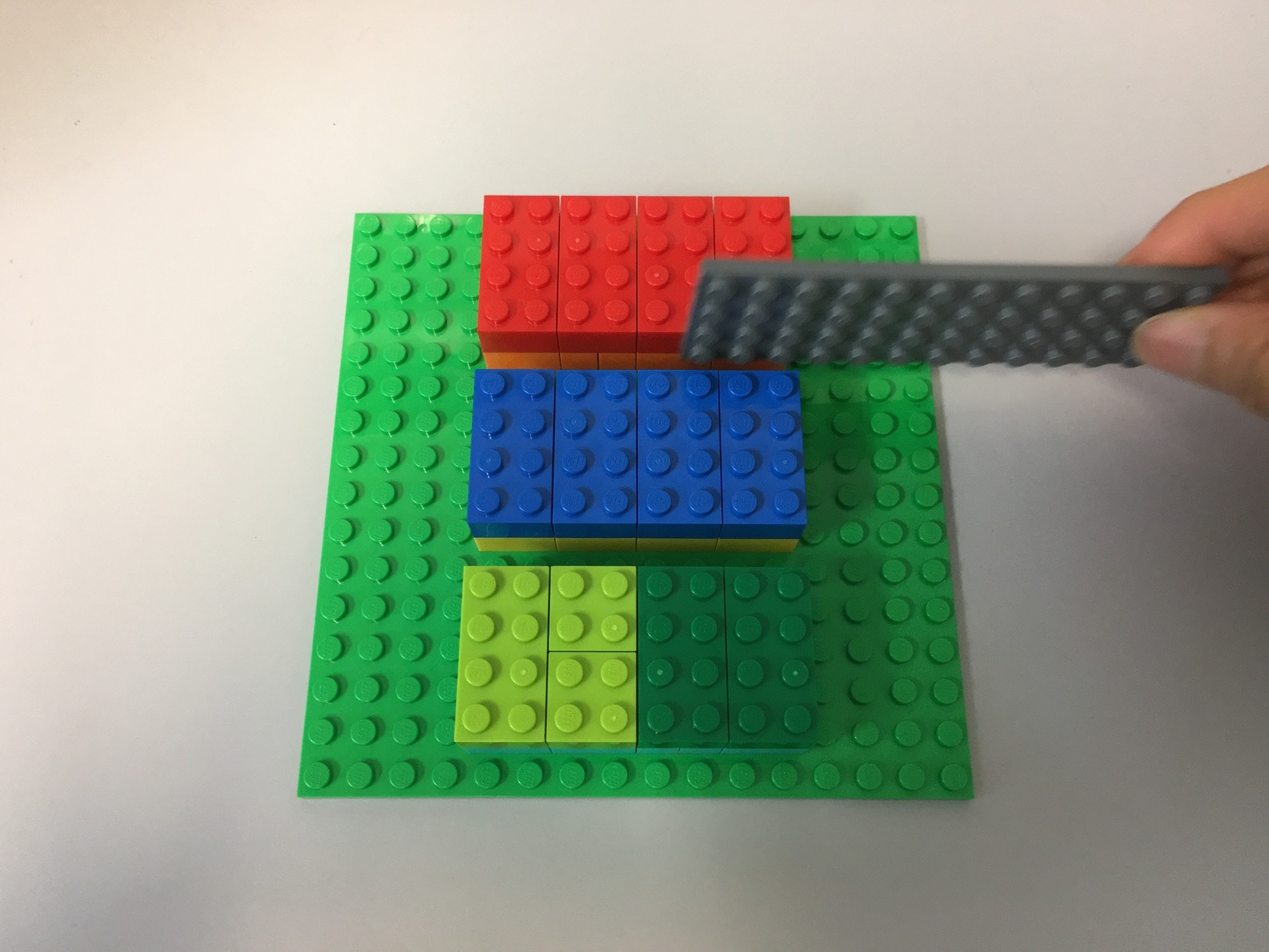Slice 0 along the first dimension returns the first big "chunk".

In [ ]:
t[0, :, :]


Slice 1 along the first dimension returns the next big "chunk".

In [ ]:
t[1, :, :]


### Slicing along the second dimension¶

The second dimension views our tensor this way:

t = np.array([[
second_level_array0,  # [  1,  -1,  11, -11],
second_level_array1   # [  2,  -2,  22, -22]   <-- note the single square brackets
surrounding each second level array [ ]
],

[
second_level_array0,  # [  3,  -3,  33, -33],
second_level_array1   # [  4,  -4,  44, -44]
],

[
second_level_array0,  # [  5,  -5,  55, -55],
second_level_array1   # [  6,  -6,  66, -66]
]])

Because this is a slice, each second-level array is actually composed of 3 vectors:

second_level_array: [[  1,  -1,  11, -11], [  3,  -3,  33, -33], [  5,  -5,  55, -55]]

second_level_array: [[  2,  -2,  22, -22], [  4,  -4,  44, -44], [  6,  -6,  66, -66]]

A slice along the second dimension will "slice the cake" horizontally: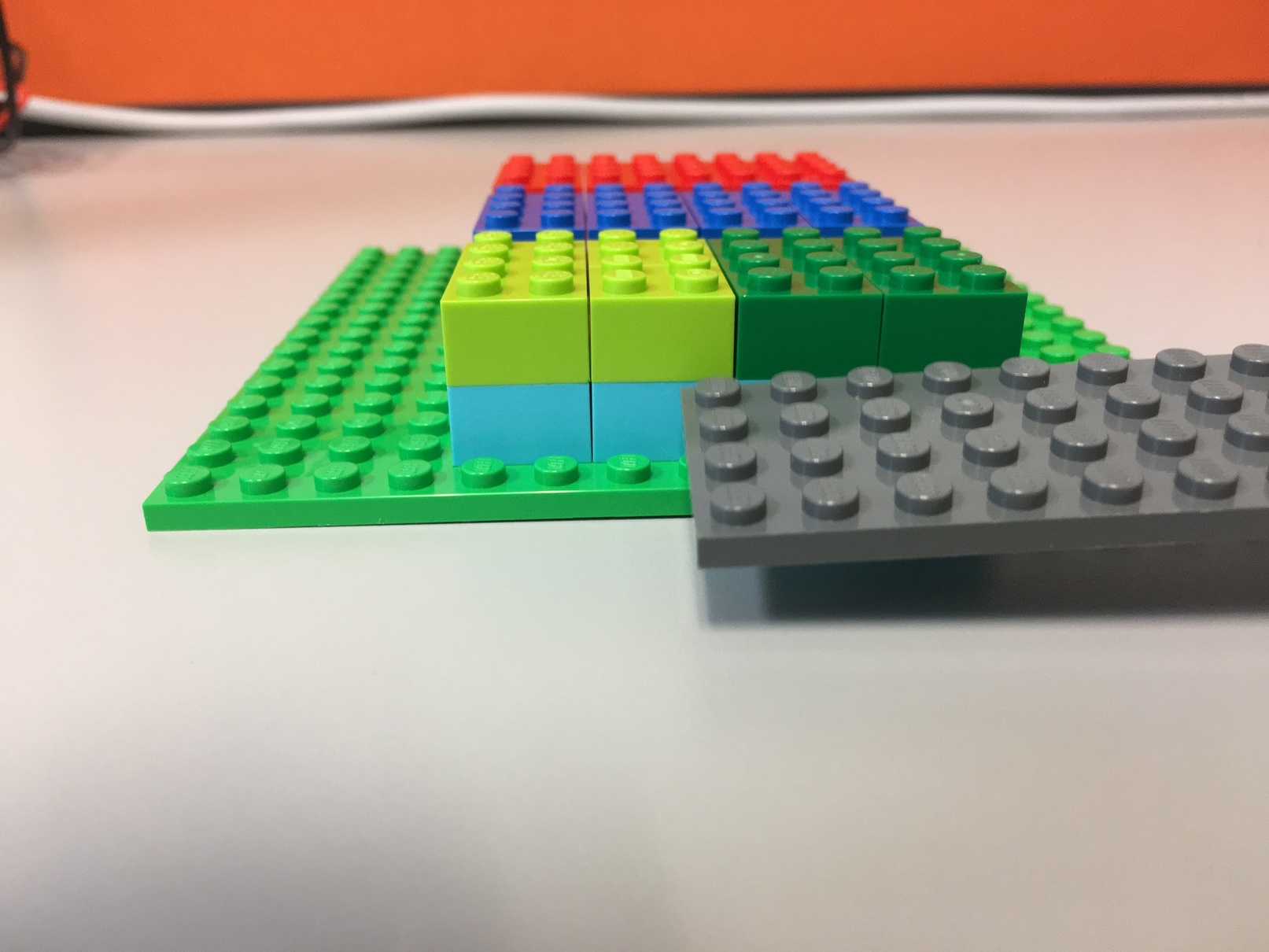Slice 0 along the 2nd dimension:

In [ ]:
t[:, 0, :]


Slice 1 along the 2nd dimension:

In [ ]:
t[:, 1, :]


### Slicing along the third dimension¶

The third dimension is the hardest to visualize, because we need to look at each element in the deepest nested array.

t = np.array([[
[element0, element1, element2, element3],  # [  1,  -1,  11, -11],
[element0, element1, element2, element3]   # [  2,  -2,  22, -22]
],

[
[element0, element1, element2, element3],  # [  3,  -3,  33, -33],
[element0, element1, element2, element3]   # [  4,  -4,  44, -44]
],

[
[element0, element1, element2, element3],  # [  5,  -5,  55, -55],
[element0, element1, element2, element3]   # [  6,  -6,  66, -66]
]])

In other words:

• the 0th slice on the third dimension creates an array that "collects" all the element0s
• the 1st slice "collects" all the element1s,
• and so on

Something like this:

third_dimension_slice: [1, 2], [3, 4]  [5, 6]

third_dimension_slice: [-1, -2], [-3, -4], [-5, -6]

Here's slicing looks like for the 3rd dimension, for the first slice: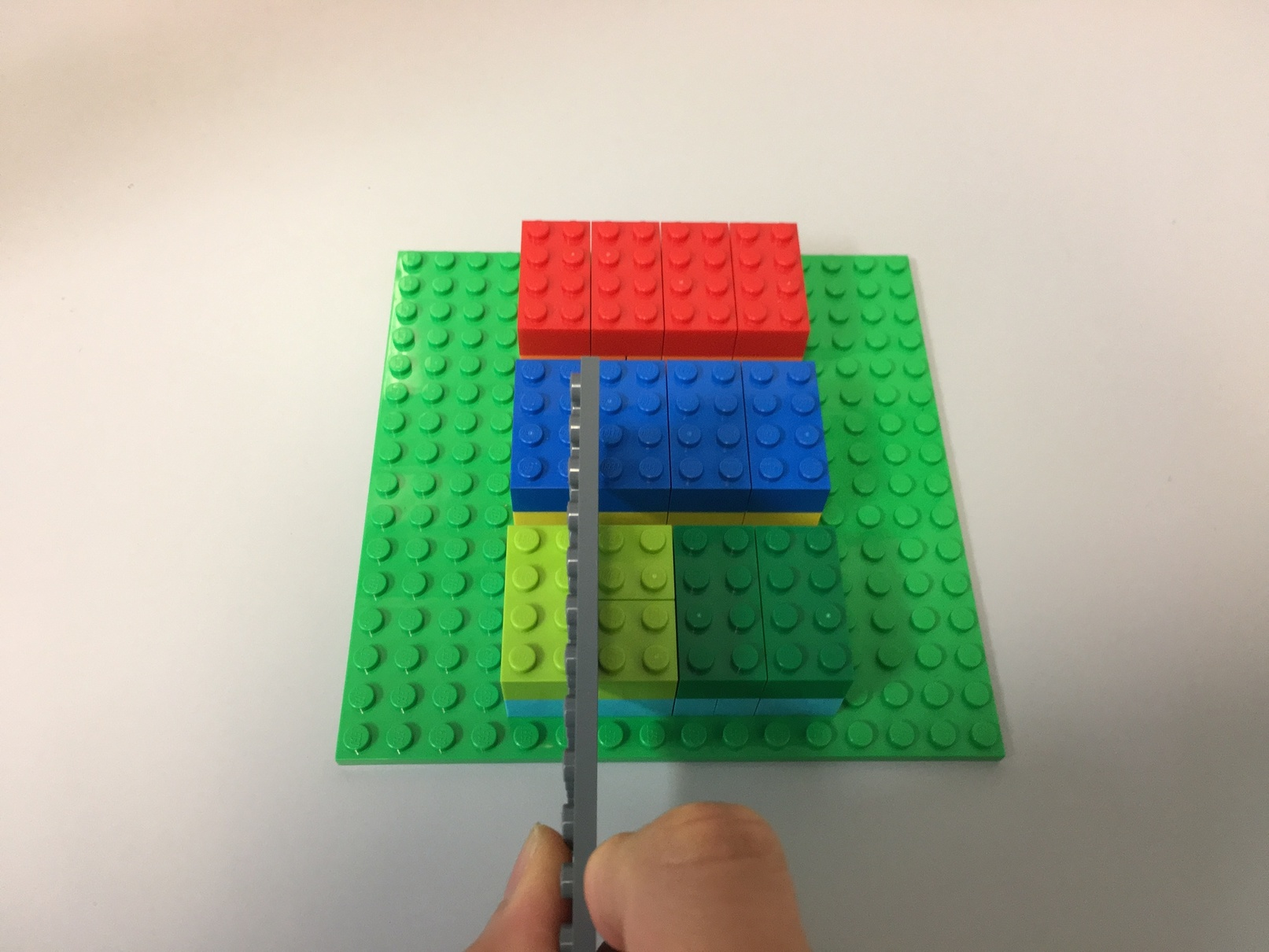In [ ]:
t[:, :, 0]

In [ ]:
t[:, :, 1]

In [ ]:
t[:, :, 2]

In [ ]:
t[:, :, 3]


## Tensor of a 2D image¶

The tensor for a 2D image (3 rows, 2 columns, 3 channels) that in channels last ordering looks like: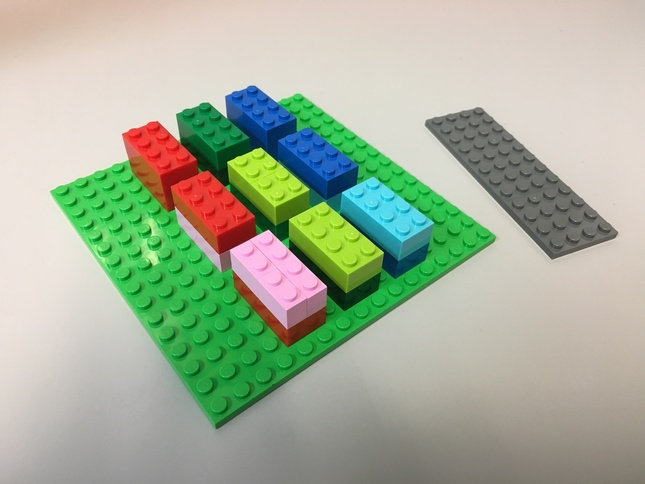### Slicing by row¶

(The 2D image is facing sideways)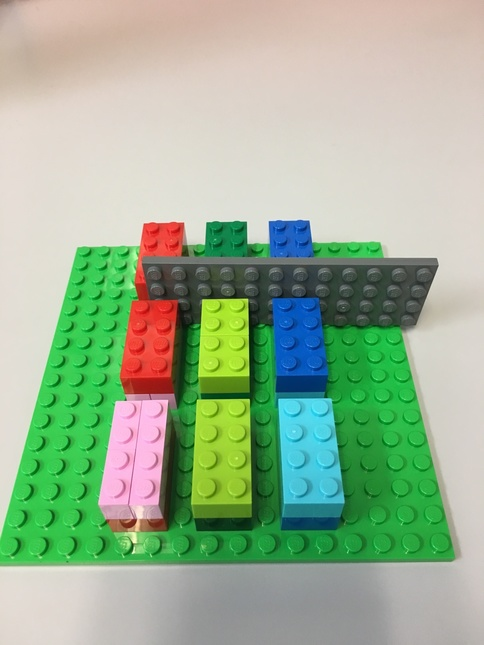### Slicing by column¶

(The 2D image is facing sideways)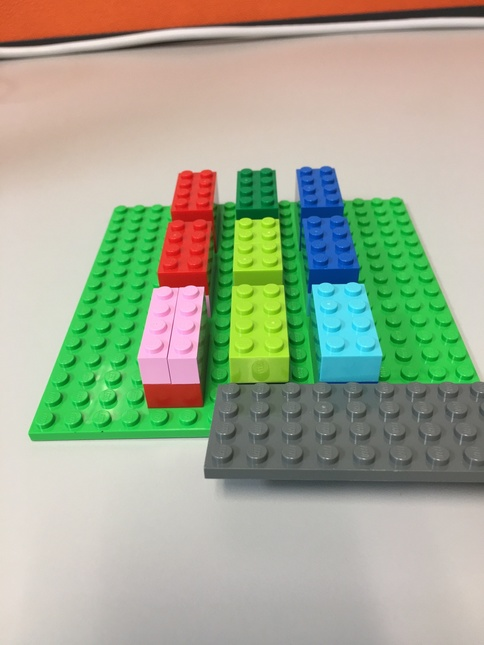### Slicing by channel¶

(The 2D image is facing sideways)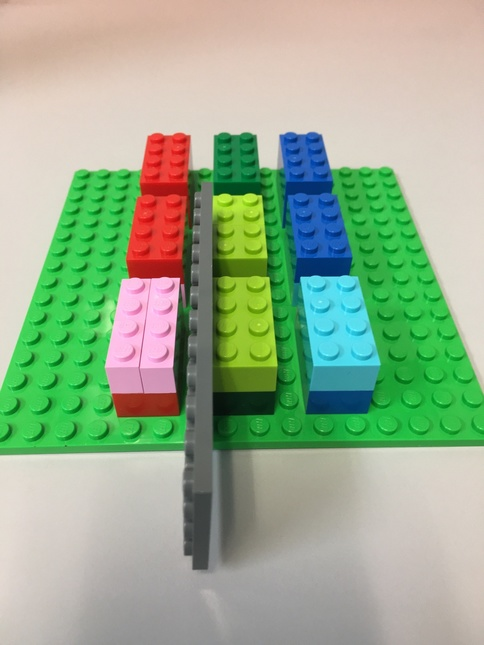# Slicing a 2D image using NumPy¶

In [ ]:
from PIL import Image
import requests
import matplotlib.pyplot as plt

url = 'https://edoras.sdsu.edu/doc/matlab/toolbox/images/colorcube.jpg'

image = Image.open(requests.get(url, stream=True).raw)


Next, we'll wrap the image in a numpy.array, which converts it to a tensor.

We'll get its shape.

In [ ]:
tensor = np.array(image)

tensor.shape


Let's also check the number of dimensions

In [ ]:
tensor.ndim # number of dimensions


Finally, plot the image

In [ ]:
plt.imshow(image)
plt.axis('off')
plt.show()


Let's get the first 10 rows

In [ ]:
tensor[:10, :, :].shape

In [ ]:
plt.imshow(tensor[:10, :, :])
plt.axis('off')
plt.show()


Let's get the first 100 columns

In [ ]:
tensor[:, :100, :].shape

In [ ]:
plt.imshow(tensor[:, :100, :])
plt.axis('off')
plt.show()


Let's get the middle 10 rows.

That's between (num_rows / 2) - 5 and (num_rows / 2) + 5 rows

In [ ]:
num_rows = tensor.shape # recall shape = (row, column, channel)
num_rows

In [ ]:
# // means divide and get the integer value, for example: 5//2 = 2

middle_ten_rows = tensor[(num_rows//2 - 5):(num_rows//2 + 5):1, :, :]

middle_ten_rows.shape

In [ ]:
plt.imshow(middle_ten_rows)
plt.axis('off')
plt.show()


Let's get the middle 10 columns.

That's between (num_cols / 2) - 5 and (num_cols / 2) + 5 rows

In [ ]:
num_cols = tensor.shape
num_cols

In [ ]:
middle_ten_cols = tensor[:, (num_cols//2 - 5):(num_cols//2 + 5):1, :]

middle_ten_cols.shape

In [ ]:
plt.imshow(middle_ten_cols)
plt.axis('off')
plt.show()


# Slicing to get per-channel data¶

This image has 3 colour channels: red, green, blue. This is known as the RGB colour space, and is the most commonly used.

An alternative colour space is blue, green, red on libraries such as OpenCV. There are converters available to convert between RGB to BGR, and other colour spaces.

Let's see how we can get the first channel (red).

Here's the syntax to get a slice

np.array[slice1, slice2, slice3, ...]

So for our 3-D tensor, we use : to denote the slice for all rows and all columns, and 0 as the index of the first channel.

In [ ]:
# all_rows, all_columns, red_channel

tensor[:, :, 0].shape

In [ ]:
# use grayscale colormap. Otherwise default is 'viridis' (see matplotlib.rcParams)
plt.imshow(tensor[:, :, 0], cmap='gray')
plt.axis('off')
plt.title('Red channel only (black: 0, white: 255)')
plt.show()

In [ ]:
# all_rows, all_columns, green_channel

tensor[:, :, 1].shape

In [ ]:
plt.imshow(tensor[:, :, 1], cmap='gray')
plt.axis('off')
plt.title('Green channel only (black: 0, white: 255)')
plt.show()

In [ ]:
# all_rows, all_columns, blue_channel

tensor[:, :, 2].shape

In [ ]:
plt.imshow(tensor[:, :, 2], cmap='gray')
plt.axis('off')
plt.title('Blue channel only (black: 0, white: 255)')
plt.show()


# Channel-first ordering¶

Our example image is using channels-last dimension ordering.

Some platforms prefer channels-first dimension ordering, where the shape is:

(channels, rows, columns)

Let's see how we can convert an image from channels-last to channels-first ordering.

Note that MatplotLib will only accept images that are channels-last ordering. It will fail to plot an image with channels-first ordering (you'll have to convert it back).

In [ ]:
tensor.shape

In [ ]:
np.moveaxis?

In [ ]:
# np.moveaxis(a, source, destination)

# move the last axis (-1) to become the first axis
np.moveaxis(tensor, -1, 0).shape


# Grayscale images¶

Grayscale images (or black and white images) have only 1 channel. So, they are 2-D tensors.

However, when you download them from the internet, they have 3 channels. This can get confusing.

In [ ]:
url = 'https://upload.wikimedia.org/wikipedia/commons/f/f2/Broadway_tower_grayscale.jpg'

image_gray = Image.open(requests.get(url, stream=True).raw)

# plot the image
plt.imshow(image_gray)
plt.axis('off')
plt.show()


The shape will show 3 channels.

In [ ]:
np.array(image_gray).shape


The values of the 3 channels are all the same.

In [ ]:
gray = np.array(image_gray)

np.testing.assert_array_equal(gray[:, :, 0], gray[:, :, 1]) # no assert means they are equal
np.testing.assert_array_equal(gray[:, :, 0], gray[:, :, 2]) # no assert means they are equal
np.testing.assert_array_equal(gray[:, :, 1], gray[:, :, 2]) # no assert means they are equal


You can pick any one of them without loss of data.

In [ ]:
plt.imshow(gray[:, :, 0], cmap='gray')
plt.axis('off')
plt.show()